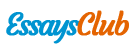# 2017 Isb Market Efficiency Price Ceiling Price Floor

Autor:   •  November 8, 2018  •  837 Words (4 Pages)  •  44 Views

Page 1 of 4

...

Price Ceiling

Price[pic 10]

[pic 11]

[pic 12]

A[pic 13]

X[pic 14][pic 15][pic 16][pic 17]

B C

PC[pic 18][pic 19]

D E F G L

PCL[pic 20]

H J K

I[pic 21]

Q QC QCL Quantity

CSComp = A + B + C, PSComp = D + E + H, SWComp = A + B + C + D + E + H

What if only Q produced?

CSCL = A + B + D if only the highest reservation price consumers are allowed to buy—less if consumers from X to PCL allowed to buy

PSCL = H

SWCL = A + B + D + H—this is a max

DWL = CSComp – CSCL C + E

What if gov’t paid the producers their costs of producing the additional QCL – Q units the demanders want at price PCL, i.e., J + K + F + G + L.

CSCL = A + B + C + D + E + F + G

PSCL = [TR] – {VC} = [H + I + J + K + (J + K + F + G + L)] – {I + J + K + L + F + G}=H+J+K

Gov’t Subsidy = F + G + J + K + L

SWCL = A + B + C + D + E + F + G + H + J + K – (F + G + J + K + L)

= A + B + C + D + E + H – L

SWComp – SWCL = A + B + C + D + E + H – (A + B + C + D + E + H – L) = L

L = DWL. The QCL – QC units produced beyond the competitive outcome are values by consumers at G + K whereas they cost G + K + L to produce. Thus the benefits (G + K) are exceeded by the costs (G + K + L) by amount L

...

Download:   txt (3.3 Kb)   pdf (45.4 Kb)   docx (12.2 Kb)
Continue for 3 more pages »
Only available on Essays.club